# Java中Integer的装箱、拆箱

#### 一、关于拆箱和装箱

int(4子节) Integer
byte(1子节) Byte
short(2子节) Short
long(8子节) Long
float(4子节) Float
double(8子节) Double
char(2子节) Character
boolean(未定) Boolean

Integer a = 100;    //装箱


int b = a;      //拆箱


public class IntegerTest {
public static void main(String[] args) {
Integer a = 100;
int b = a;
}
}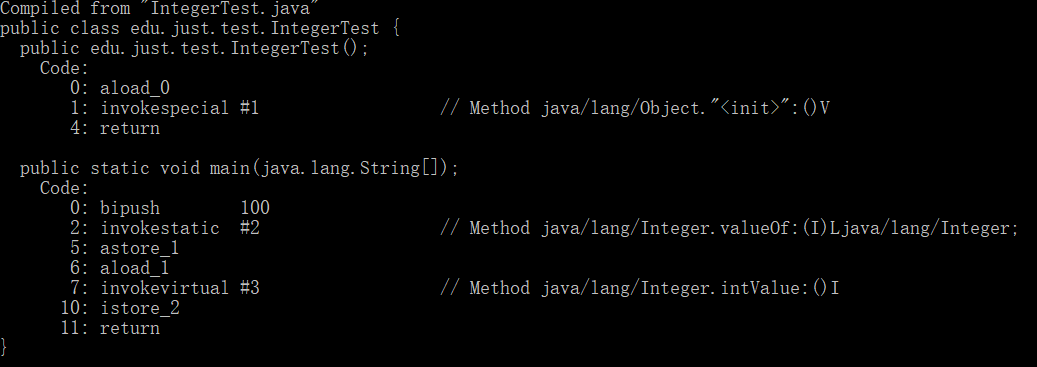public class IntegerTest {
public static void main(String[] args) {
Double d = 200d;
double f = d;

Short aa = 1;
short bb = aa;

Long l = 10000000l;
long ll = l;
}
}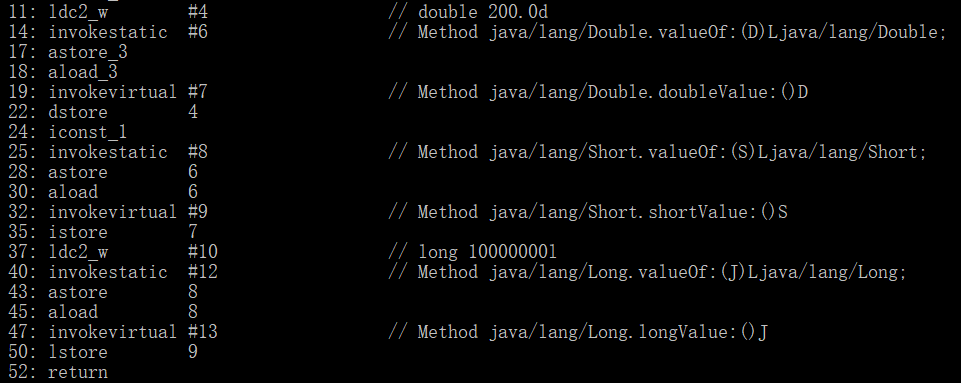#### 二、关于 Integer.valueOf

public static Integer valueOf(int i) {
if (i >= IntegerCache.low && i <= IntegerCache.high)
return IntegerCache.cache[i + (-IntegerCache.low)];
return new Integer(i);
}


IntegerCache 的源码如下

private static class IntegerCache {
static final int low = -128;
static final int high;
static final Integer cache[];

static {
// high value may be configured by property
int h = 127;
String integerCacheHighPropValue =
sun.misc.VM.getSavedProperty("java.lang.Integer.IntegerCache.high");
if (integerCacheHighPropValue != null) {
try {
int i = parseInt(integerCacheHighPropValue);
i = Math.max(i, 127);
// Maximum array size is Integer.MAX_VALUE
h = Math.min(i, Integer.MAX_VALUE - (-low) -1);
} catch( NumberFormatException nfe) {
// If the property cannot be parsed into an int, ignore it.
}
}
high = h;

cache = new Integer[(high - low) + 1];
int j = low;
for(int k = 0; k < cache.length; k++)
cache[k] = new Integer(j++);

// range [-128, 127] must be interned (JLS7 5.1.7)
assert IntegerCache.high >= 127;
}

private IntegerCache() {}
}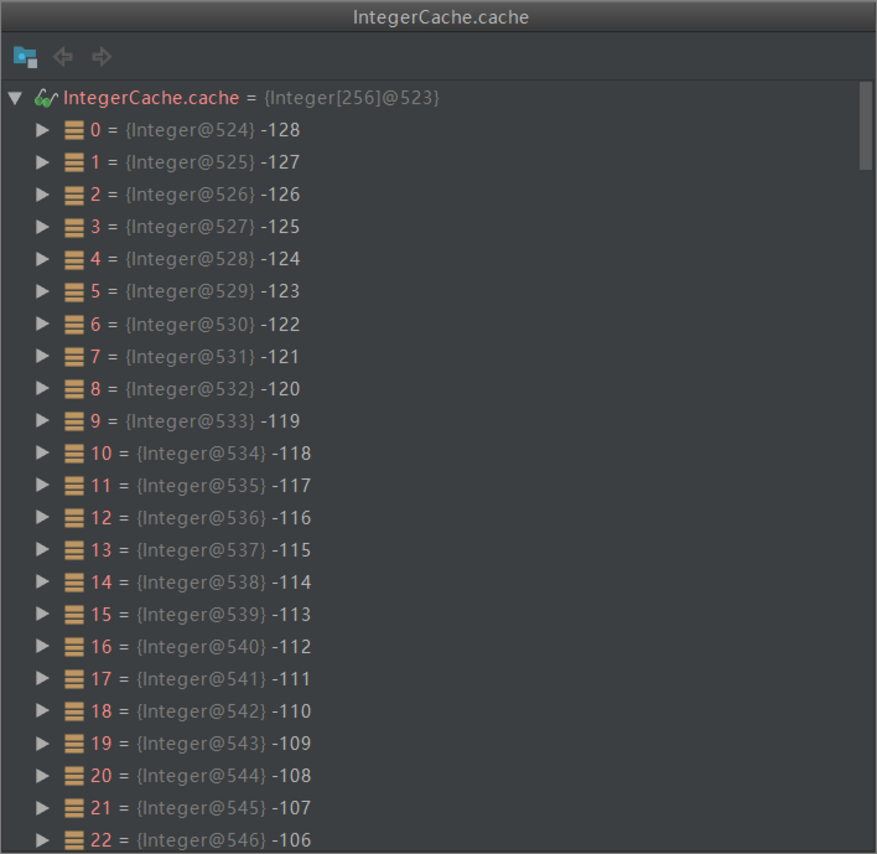#### 三、相关面试题

1.输出以下结果

Integer a = 100;
Integer b = 100;
Integer a1 = 200;
Integer b1 = 200;
Integer a2 = new Integer(100);

a == b;
a1 == b1;
a == a2;


a 和 b 先各自调用 Integer 的 valueOf() 方法进行装箱，因为数值 a 和 b 的数值是100的，在 [-128，127] 这个范围之间，所以执行 Integer a = 100 这行代码的时候，在装箱的时候，会先初始化一个长为 255 的 的数组，里面的值的范围是 -128~127，变量 a 的值直接从 cache 对应的位置拿，之后执行到 Integer b = 100 的时候，便去这个已经实例化好了的数组中拿对应位置的值，该位置和变量 a 在数组中的位置相同，最终，这两个变量拿到的是同一个数组的同一个位置的变量，此时肯定是相同的

a 和 b 的数值为200时，在调用 valueOf() 方法进行装箱的时候，因为值不在 [-128，127] 间，因此没有实例化 cache 数组和从数组中拿值的过程，因此只能各自实例化一个 Integer 的对象，此时这两个对象的地址是不同，因此不同

a 和 a2 比较的时候，由于 a 调用 valueOf() 进行自动装箱，而 a2 已经是 Integer 对象，相当于两个不同对象之间的比较，因为地址不同，所以肯定不等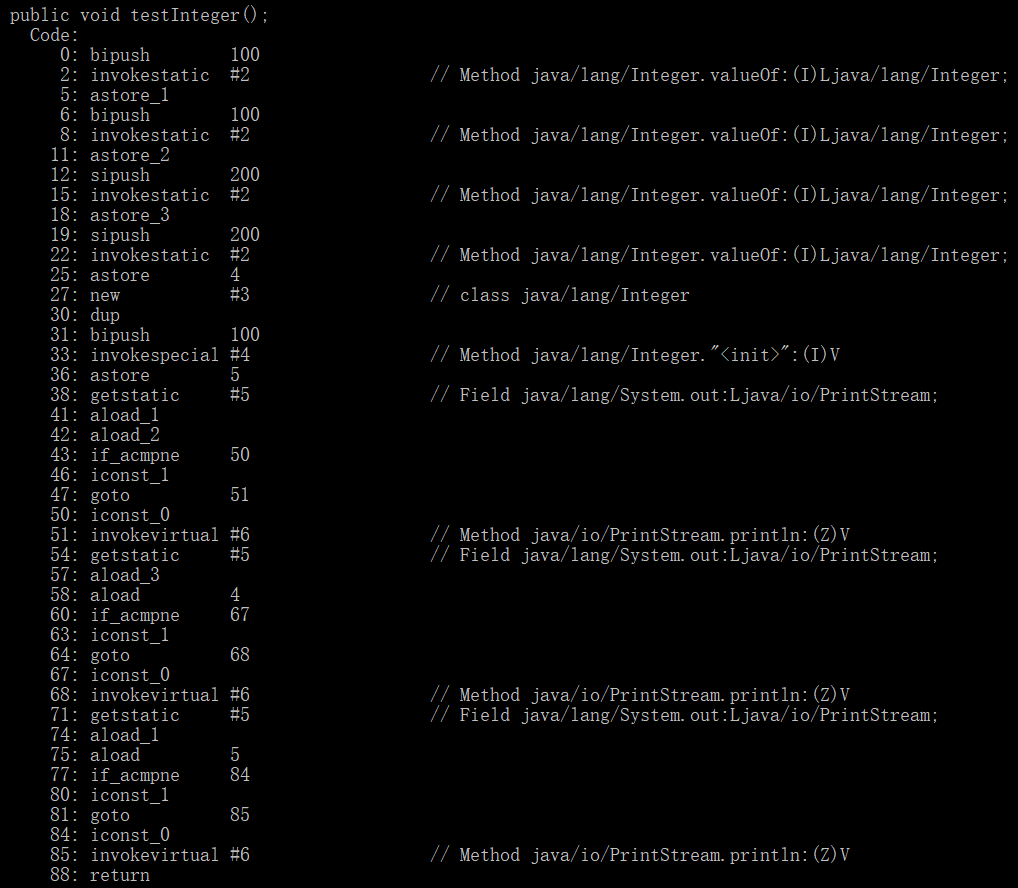2.输出以下代码运行的结果

Double i1 = 100.0;
Double i2 = 100.0;
Double i3 = 200.0;
Double i4 = 200.0;

i1 == i2
i3 == i4


public static Double valueOf(double d) {
return new Double(d);
}

public Double(double value) {
this.value = value;
}


##### PS

3.输出以下代码运行的结果

Boolean b1 = false;
Boolean b2 = false;
Boolean b3 = true;
Boolean b4 = true;

b1 == b2
b3 == b4
b1 == b3


public static Boolean valueOf(boolean b) {
return (b ? TRUE : FALSE);
}


public static final Boolean TRUE = new Boolean(true);

public static final Boolean FALSE = new Boolean(false);


4.输出以下代码运行的结果

Integer a = 10;
Integer b = 20;
Integer c = 30;
Integer d = 30;
Integer e = 300;
Integer f = 300;
Integer g = a + b;

c == d;
e == f;
c == g;
c == (a + b);
c.equals(a + b);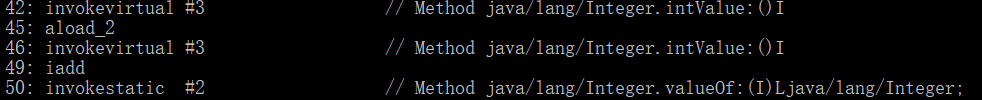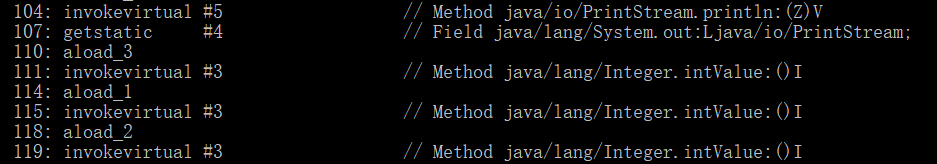a + b 的时候，a 和 b 各自执行 intVal() 方法进行拆箱，完成相加的计算之后，再对计算出的结果执行 valueOf() 方法进行装箱，此时再传入 equals 方法进行比较。equals 比较两个对象的时候，直接比较两个值拆箱之后的结果，即比较的是数值，本例中两个数值相同，所以输出为 true

public boolean equals(Object obj) {
if (obj instanceof Integer) {
return value == ((Integer)obj).intValue();
}
return false;
}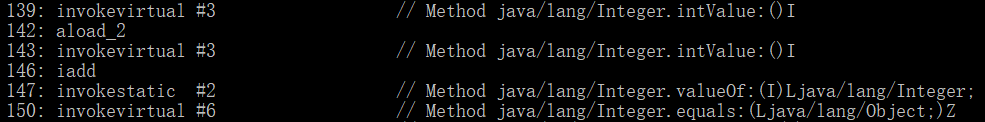5.输出以下代码运行的结果

Integer a = 10;
Integer b = 20;
Long g = 30L;
Long h = 20L;

g == (a + b);
g.equals(a + b);
g.equals(a + h);


#### 四、参考